• 闽南网首页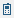手机闽南网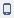新闻客户端

• 2015年全国各地
高考试题及答案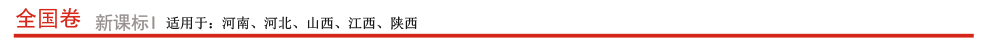（试题+答案）

（试题+答案）

（试题+答案）

（试题+答案）

（试题+答案）

（试题+答案）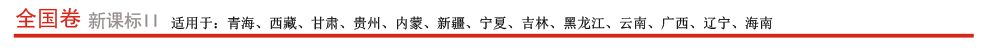（试题+答案）

（试题+答案）

（试题+答案）

（试题+答案）

（试题+答案）

（试题+答案）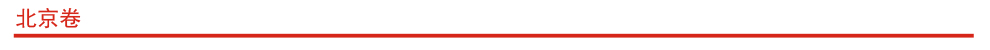（试题+答案）

（试题+答案）

（试题+答案）

（试题+答案）

（试题+答案）

（试题+答案）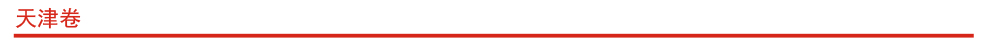（试题+答案）

（试题+答案）

（试题+答案）

（试题+答案）

（试题+答案）

（试题+答案）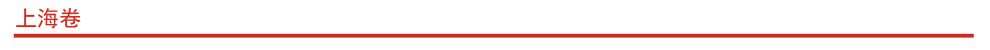语文
（试题+答案）

文数
（试题+答案）

理数
（试题+答案）

英语
（试题+答案）

政治
（试题+答案）

地理
（试题+答案）

历史
（试题+答案）

物理
（试题+答案）

化学
（试题+答案）

生物
（试题+答案）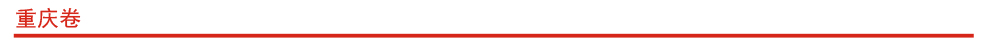（试题+答案）

（试题+答案）

（试题+答案）

（试题+答案）

（试题+答案）

（试题+答案）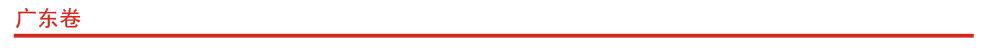（试题+答案）

（试题+答案）

（试题+答案）

（试题+答案）

（试题+答案）

（试题+答案）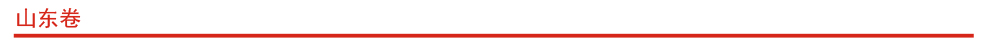（试题+答案）

（试题+答案）

（试题+答案）

（试题+答案）

（试题+答案）

（试题+答案）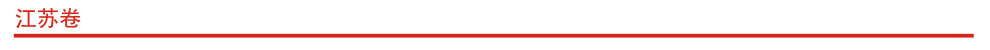（试题+答案）

（试题+答案）

（试题+答案）

（试题+答案）

（试题+答案）

（试题+答案）

（试题+答案）

（试题+答案）

（试题+答案）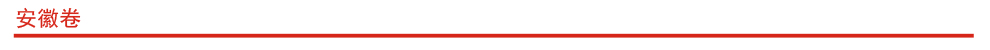（试题+答案）

（试题+答案）

（试题+答案）

（试题+答案）

（试题+答案）

（试题+答案）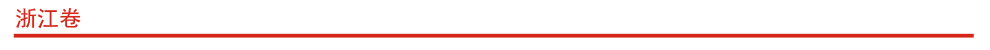（试题+答案）

（试题+答案）

（试题+答案）

（试题+答案）

（试题+答案）

（试题+答案）

（试题+答案）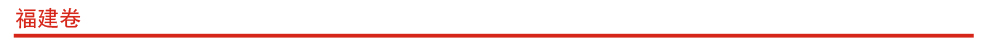（试题+答案）

（试题+答案）

（试题+答案）

（试题+答案）

（试题+答案）

（试题+答案）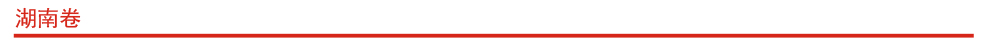（试题+答案）

（试题+答案）

（试题+答案）

（试题+答案）

（试题+答案）

（试题+答案）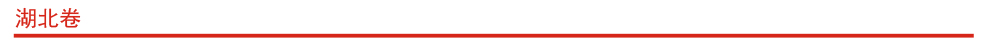（试题+答案）

（试题+答案）

（试题+答案）

（试题+答案）

（试题+答案）

（试题+答案）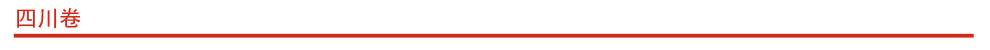（试题+答案）

（试题+答案）

（试题+答案）

（试题+答案）

（试题+答案）

（试题+答案）

电话:0595-28679111 传真:0595-22567376 地址:福建省泉州市泉秀街沉洲路莲花大厦4楼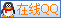CopyRight 2012 闽南网(海峡都市报闽南版报社主办) 版权所有 闽ICP备10206509号 闽互联网新闻信息服务备20111002号